Sie sind hier: ICP » R. Hilfer » Publikationen

# 3 Results

[page 4, §1]
[4.1.1] It follows from the general results in Ref.  that the first model defined by eqs. (6) and (9) is equivalent to the fractional master equation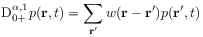(10)

with intitial condition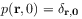(11)

and fractional transition rates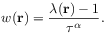(12)

Here the fractional time derivative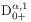of order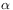and type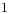in eq. (10) is defined as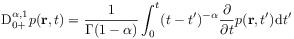(13)

thereby giving a more precise meaning to the symbolic notation in eq. (1).

[page 5, §1]    [5.1.1] The result is obtained from inserting the Laplace transform of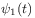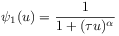(14)

and the Fourier transform of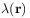, the so called structure function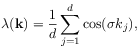(15)

into eq. (5). [5.1.2] This gives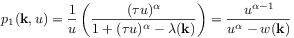(16)

where the Fourier transform of eq. (12) was used in the last equality and the subscript refers to the first model. [5.1.3] Equation (16) equals the result obtained from Fourier-Laplace transformation of the fractional Cauchy problem defined by equations (10) and (11). [5.1.4] Hence a CTRW-model withand the fractional master equation describe the same random walk process in the sense that their fundamental solutions are the same.

[5.2.1] The continuum limit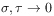was the background and motivation for the discussion in Ref. . [5.2.2] It follows from eq. (1.9) in Ref.  by virtue of the continuity theorem  for characteristic functions that for the first model the continuum limit with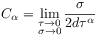(17)

leads for all fixed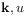to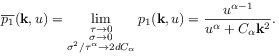(18)

[5.2.3] Here the expansion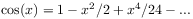has been used. [5.2.4] Therefore the solution of the first model with waiting time densityconverges in the continuum limit to the solution of the fractional diffusion equation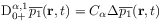(19)

with initial condition analogous to eq. (11).

[5.3.1] Consider now the second model with waiting time density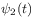given by eq. (8). [5.3.2] In this case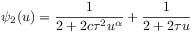(20)

and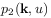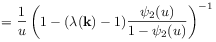(21)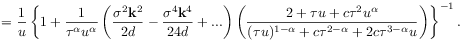From this follows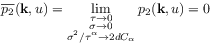(22)

showing that the continuum limit as in eq. (17) with finite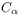does not give rise to the propagator of fractional diffusion. [5.3.3] On the other hand the conventional continuum limit with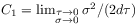exists and yields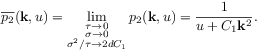(23)

the Gaussian propagator of ordinary diffusion with diffusion constant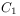.